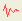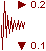## Search Results

1. Select Seismic Loadingfrom the Loading menu. Note: you can also right-click on the Stress Block / Seismic Load icon and select Seismic Loading from the popup menu.
2. In the Seismic Load dialog, enter seismic coefficients for the Horizontal and / or Vertical directions. You may also select the stage at which the seismic force is applied. select [OK].

## Seismic Coefficients

The Seismic Coefficients are dimensionless coefficients which represent the (maximum) earthquake acceleration as a fraction of the acceleration due to gravity. Typical values are in the range of 0.1 to 0.3.

When a seismic coefficient is defined, an additional Body Force will be applied to each finite element in the mesh, as follows:

Seismic Force = Seismic Coefficient * Body Force (due to gravity)

= Seismic Coefficient * (area of element * Unit Weight of element material)

• Body Force (due to gravity) is simply the self-weight of a finite element.
• The Seismic body force is vectorially added to the (downward) Body Force which exists due to gravity, to obtain the total body force acting on the element.

NOTE: acceleration due to gravity (g = 9.81 m/s2) is already incorporated into the Unit Weight of Material (remember that the dimensions of Unit Weight are force/volume), and therefore does not explicitly appear in the above equation. The Unit Weight of material is the Unit Weight which is defined for each material in the Define Material Properties dialog, when Body Force is included with the Initial Element Loading (see below).

If Body Force is NOT included in the Initial Element Loading, then the applied Seismic Force will be ZERO, even if seismic coefficients are defined in the Seismic Load dialog. You must remember this if you want to use Seismic Load in combination with Constant Field Stress, since by default, the Initial Element Loading for Constant Field Stress is Field Stress Only.

Direction Convention

The following direction convention is used for the seismic coefficients in RS2:

• a POSITIVE Horizontal coefficient represents a force applied to the RIGHT.
• a NEGATIVE Horizontal coefficient represents a force applied to the LEFT.
• A POSITIVE Vertical coefficient represents a force applied UPWARDS.
• A NEGATIVE Vertical coefficient represents a force applied DOWNWARD.

When carrying out a finite element analysis with Seismic Loading, you should run multiple analyses using different combinations and directions of Horizontal and Vertical seismic coefficients, in order to evaluate the effect of seismic force direction on the analysis results (e.g. stresses, displacements, strength factor etc).

## Application of Seismic Coefficient

The Application of Seismic Coefficient option allows you to specify the stage at which the seismic load is applied. This is only applicable for multi-stage models (i.e. Number of Stages > 1).

Applied in Stage

The Applied in Stage option allows you to select the stage at which the seismic load will be applied. Strictly speaking, the seismic load should be applied at the FINAL stage of excavation. If seismic load is applied at an intermediate stage of excavation, the effect of the load will propagate to all subsequent stages (i.e. the seismic load is NOT removed at subsequent stages, after the initial stage of application).

NOTE: if you are using the Load Split option, this does NOT have any effect on the seismic force. The seismic force which is applied will always equal the element weight multiplied by the seismic coefficient. Load split factors are NOT applied to the seismic force, if you are using the Applied in Stage option and the Load Split option.

Applied when body force is applied

The Applied when body force is applied option will automatically apply the seismic load at the stage when the body force of an element (material) is first applied. Generally this will be the first stage. Furthermore, the seismic load will be applied according to the load split, if the Load Split option is in use. This means that the seismic load will be applied in successive stages, according to the staging of the load split factors which are applied to the body force.

This option only exists to maintain backward compatibility with RS2 version 4.0. The use of this option is NOT recommended.